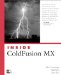# For Loop

The For loop is the CFScript form of the CFLOOP tag shown in the following code:

` <cfloop INDEX="i" FROM="1" TO="10">  <cfset array[i] = i>  </cfloop> `

This will set the values of the elements 1 10 in an array to the value of its index. In CFScript, it would look like the following:

` <cfscript>      for(i=1; i LTE 10; i = i + 1){           array[i] = i;      }  </cfscript> `

The general form of a For loop in CFScript is as follows:

` For (starting expression; conditional expression; incremental expression) `

The starting expression sets the values of the index to be used within the loop (i=1 in the preceding example), and the conditional expression is the condition that must be maintained for the loop to continue. When the conditional expression is no longer true, the loop terminates. The incremental expression defines the manner in which to modify the index. This could be something like the preceding example; it could be i = i + 1, which increments the value of i every time through the loop, or something like i = i + 2 to loop over only odd or only even numbers, depending on the initial setting.

Note

Unlike ECMA Script and JavaScript, CFScript supports all the CFML expressions. This includes operators (such as +, -, =, and so on), as well as all CFML functions. You must use CFML operators, such as LT, GT, and EQ. You cannot use JavaScript operators, such as <, >, ==, or ++, so be careful.

#### For-In

Another application of the For loop is the ability to loop over structures. Let's create a structure of pet information:

` <cfscript>      pet_struct = StructNew();      pet_ struct.cat = "Asterix";      pet_ struct.cat = "Jenghis";      pet_ struct.cat = "Newt";      pet_ struct.dog = "Delilah";      pet_ struct.bird = "Peekaboo";  </cfscript> `

We can then loop over the pets using CFLOOP:

` <cfloop COLLECTION="#pet_ struct #" ITEM="pet">  <cfoutput>#pet#</cfoutput></br>  </cfloop> `

This will print the names of the pets. In CFScript, we can do the same thing:

` <cfscript>      for(pet in pet_ struct){          WriteOutput("#pet#</br>");      }  </cfscript>  `

#### while

Yet another type of loop is what is known as the pretest, or While, loop. What this means is that given a condition, the condition must be true before the rest of the loop will be executed. This loop is useful where a group of statements will not always need to be executed. Take for example the case of a shopping cart. If you want to loop over the contents of the cart, the following loop might be useful:

` <cfscript>      //Set the number of the item to display      index = 1;      //While loop      while(index LTE cart_size){          //Output the item at the specified index          WriteOutput(shopping_cart[index]);          //Increment index          index = index + 1;      }  </cfscript> `

In the preceding example, we are using index to denote the current item to be printed. The while loop is contingent upon the index being less than the number of items in the cart, which is contained in cart_size. If the size of the cart is 0, the loop will not execute and no items will be displayed. If the cart_size is greater than 0, all items in the cart will be displayed.Inside Coldfusion MX
ISBN: 0735713049
EAN: 2147483647
Year: 2005
Pages: 579